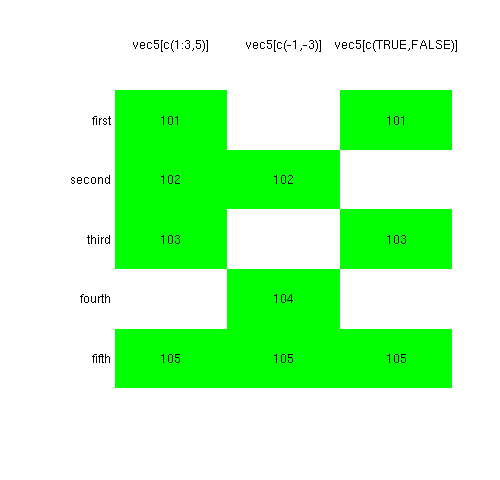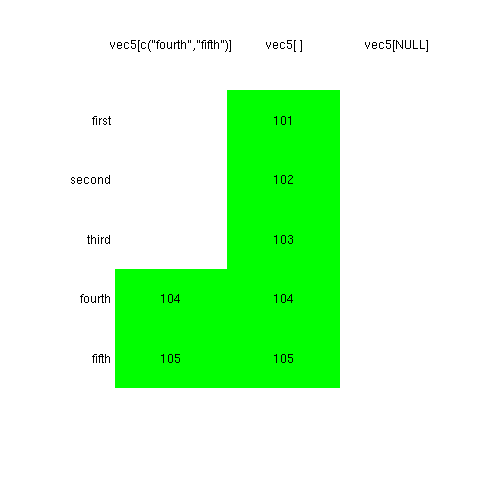01 Jan

# More R Subscript

## Vector

Figures 1 and 2 show the results of six cases of subscripting a particular vector that can be defined with:

```vec5 <- c(first=101, second=102, third=103, fourth=104,
fifth=105)```

This particular vector is numeric, but subscripting is the same no matter what type of vector it is.

Figure 1Figure 2We discuss each of these in more detail.

### Positive numbers

When you subscript with positive numbers, you are saying which elements you want to keep.

There are two ways in which Figure 1 is misleading for this case:

• the order need not be the same as the original.

For example

``` > vec5[3:1]
third second  first
103    102    101```

is different from

``` > vec5[1:3]
first second  third
101    102    103```
• subscripts can be repeated.
``` > vec5[c(1,4,4,5,2,1,4)]
first fourth fourth  fifth second  first fourth
101    104    104    105    102    101    104```

### Negative numbers

When you subscript with negative numbers, you are saying which elements you don’t want to keep. The order of elements in the resulting vector is always the same as in the original — there are just some elements gone.

### Logical values

When you subscript with a logical vector, you are selecting the elements that correspond to `TRUE`. Unlike in Figure 1, this is often something like:

``` > vec5[ vec5 > 103 ]
fourth  fifth
104    105```

That is, the logical vector doing the subscripting is the same length as the original vector, and it is the result of some comparison operation.

A logical subscript is similar to a negative number subscript. They both leave the elements of the result in the same order as the original with some of the elements not there.

The example in Figure 1 is actually rather weird. Since the subscripting vector is shorter than the original vector, it is replicated to have the same length as the original. Such recycling is either handy or dangerous, depending on the context.

### Character values

Subscripting with a character vector is just the same as subscripting with positive numbers. The difference is that you are specifying the elements to select using the names of the vector rather than the order within the vector.

```> vec5[c("fourth", "third", "fourth")]
fourth  third fourth
104    103    104```

This correspondence means that the order of the result need not be the same as in the original, and there can be repeated elements.

### Missing

If there is no argument inside the square brackets, then all elements of the original vector are selected. This seems silly. But when we get to matrices and data frames, we’ll see that it is actually very useful.

### Zero length

While it might seem like putting `NULL` inside the square brackets would be the same as putting nothing there, the results couldn’t be more different.

Subscripting with any zero length object produces a zero length result.

```> vec5[]
first second  third fourth  fifth
101    102    103    104    105
> vec5[NULL]
named numeric(0)```

## Matrix and Data Frame

A matrix and a data frame are subscripted exactly the same. A vector is conceptually a one-dimensional object while matrices and data frames are two-dimensional. So you need two subscripts for matrices and data frames. The rows and the columns are subscripted independently of each other, with a comma separating the subscripts. Here are some examples, where `twodim `can be either a matrix or a data frame:

```> twodim[1:3, 4:5]    # rows 1, 2, 3, and columns 4, 5
> twodim[1:3, "id"]   # rows 1, 2, 3, and column called "id"
> twodim[1:3, ]       # rows 1, 2, 3, and all columns```

When characters are used as subscripts, this will be referring to the row names or the column names.

### Drop

If you subscript a matrix so that it has one row or one column, then the result is not a matrix — it is a plain vector. You can ensure that the result is a matrix by adding a `drop=FALSE` to the subscripting:

```> mat[1:3, 4] # plain vector, not matrix
> mat[3, 2:5] # plain vector, not matrix
> mat[1:3, 4, drop=FALSE] # 3 by 1 matrix
> mat[3, 2:5, drop=FALSE] # 1 by 4 matrix```

If you subscript only one column of a data frame, then the default behavior is to not get a data frame. That is almost always what you want. If not, then you can use the `drop` argument to subscripting as with matrices. If you subscript a single row of a data frame, you get a data frame.

## List

You subscript lists just like vectors. Lists are vectors in the sense that counts for subscripting. Subscripting is the same no matter what type of vector it is.

There is another type of subscripting with lists — using double square brackets — that selects one component of the list.

For example

``` > examp[]
  1  2  3  4  5  6  7  8  9 10```

selects the first component of the list. That is different from

``` > examp
\$A
  1  2  3  4  5  6  7  8  9 10```

which returns a list with one component.

The difference is a little bit subtle. But only a little bit.If  a list is a train of boxcars, then `train` is the first boxcar and `train[]` is what is inside the first boxcar.  (I’m so jealous that I didn’t think of this analogy.)

The double bracket is going down inside and pulling out a piece. That piece might be a list, but it doesn’t have to be.

Let’s create a list and experiment.

``` > mylist <- list(lower=letters[1:4], upper=LETTERS[-1:-20])
> mylist[]
 "a" "b" "c" "d"
> mylist
\$lower
 "a" "b" "c" "d"
> mode(mylist[])
 "character"
> mode(mylist)
 "list"```

A way to remember it is that subscripting with a single bracket can have length greater than one, so the result has to be a list. The result of the double bracket subscripting has whatever mode that particular component of the list has.

The example above gave a hint of another form of subscripting — the use of the `\$` operator.

``` > mylist\$lower
 "a" "b" "c" "d"```

This takes the name of the list on the left and the name of the component you want on the right. The result is the component.

The `\$` operator and the double square bracket are very similar. All of the following are doing the same thing:

``` > mylist[["lower"]]
> mylist\$lower
> whichComp <- "lower"
> mylist[[whichComp]]```

A difference between the `\$` operator and double square brackets is that double square brackets can be used on all types of vectors, but `\$` is specific to lists.

## Replacement

The discussion above only talks about extraction. However, if the subscripting is on the left of an assignment, then the values are replaced. That is, the original object has some of its elements modified.

For example

` > vec5[ vec5 > 103 ] <- 103`

changes `vec5` so that 103 is its maximum value.

## Matrix and Array

Matrices are actually a special case of arrays — a matrix is a two dimensional array. An array can have any dimension (that fits on the machine).

When you subscript an array, you need to have as many subscripts as there are dimensions in the array. So you might subscript a three-dimensional array like:

` > threeD[30:40, c("G", "E", "T"), something > 0]`

The “need” just above is a lie. You can also give just one subscript. There are two ways of giving just one subscript:

• a vector
• a matrix

### Vector subscript

An array (including a matrix) is really just one long vector that has a `dim` attribute that says how to wrap its values into a higher dimensional shape.

You are free to subscript as if the object were a plain vector.

There is a danger that you can do this accidentally. For example, you might say

` > myMatrix[30:40]`

when you mean to say

` > myMatrix[30:40, ]`

The difference in the command is tiny, the difference in the result can be dramatic.

You can also subscript a data frame with a single vector, but the result is much different than with a matrix. A data frame is really a list with a component for each column. So subscripting a data frame with a single vector means you are selecting columns.

You can subscript an n-dimensional array with a matrix that has n columns. Each row contains the indices in each dimension for the location to be subscripted. The result is a vector with length equal to the number of rows in the subscripting matrix.

In modern versions of R the subscripting matrix can be either positive numbers or character. However, in older versions only numeric subscripting is supported.

Suppose that we have a matrix called `Mat` and we want the value from a random column for each row. (Why would we want to do that? I don’t know either.) The following command does that:

```Mat[cbind(1:nrow(Mat),
sample(1:ncol(Mat), nrow(Mat), replace=TRUE))]```

## Resources

Circle 8 of The R Inferno contains some ways to be fooled when subscripting.